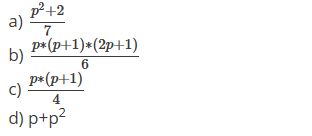# Welcome to the Induction and Recursion MCQs Page

Dive deep into the fascinating world of Induction and Recursion with our comprehensive set of Multiple-Choice Questions (MCQs). This page is dedicated to exploring the fundamental concepts and intricacies of Induction and Recursion, a crucial aspect of Discrete Mathematics. In this section, you will encounter a diverse range of MCQs that cover various aspects of Induction and Recursion, from the basic principles to advanced topics. Each question is thoughtfully crafted to challenge your knowledge and deepen your understanding of this critical subcategory within Discrete Mathematics.

Check out the MCQs below to embark on an enriching journey through Induction and Recursion. Test your knowledge, expand your horizons, and solidify your grasp on this vital area of Discrete Mathematics.

Note: Each MCQ comes with multiple answer choices. Select the most appropriate option and test your understanding of Induction and Recursion. You can click on an option to test your knowledge before viewing the solution for a MCQ. Happy learning!

### Induction and Recursion MCQs | Page 1 of 3

Q1.
By induction hypothesis, the series 1² + 2² + 3² + … + p² can be proved equivalent to ____________a.

a

b.

b

c.

c

d.

d

Q2.
What is the base case for the inequality 7ⁿ > n³, where n = 3?
Q3.
In the principle of mathematical induction, which of the following steps is mandatory?
Q4.
For m = 1, 2, …, 4m+2 is a multiple of ________

a.

3

b.

5

c.

6

d.

2

Q5.
For any integer m>=3, the series 2+4+6+…+(4m) can be equivalent to ________
Q6.
For every natural number k, which of the following is true?
Q7.
For any positive integer m ______ is divisible by 4.
Q8.
According to principle of mathematical induction, if P(k+1) = m⁽ᵏ⁺¹⁾ + 5 is true then _____ must be true.
Answer: (b).P(k) = m⁽ᵏ⁾ + 5
Q9.
Which of the following is the base case for 4ⁿ⁺¹ > (n+1)² where n = 2?